# 3. Structuring Phenoestetic Video

## 3. Structuring Phenoestetic Video

The video that I called phenoestetic is constituted by pure video streams coming from a camera placed and, one would say, forgotten, in a place, as is the case with surveillance cameras, web cameras, or, in general, any instance of voyeurism in which the imperative to look at "reality as it is"  is more categorical than that of constructing a structured cinematic speech.

The problems of the clash between the syntagm of the video and the paradigm of the interface, and of that between transparent and opaque screens, are, in these cases, even more pronounced than in semiotic video for, in that case, one had a sequence of anchors (the scenes and cuts) which could be presented in a paradigmatic way on an opaque screen and, at the user's whim, explored syntagmatically on a transparent screen.

In phenoestetic one is in the presence of an infinite, unstructured flow of images that, if no suitable organizing principle is found, can only be experienced by endless and continuous observation. This is, of course, unsatisfactory, not only because the endless observation of a video stream clashes with the more interactive attitude of multimedia but because, more simply, in these video most of the time there is absolutely nothing of the remotest interest to observe: the points of interest are few and apart.

There is—one would say—a generalized consensus that in the absence of a syntax like that of the montage, the organization of this type of video should proceed along semantic lines, that is, by identifying nuclei of meaning in the video sequence, and by organizing the video into syntagmatic units around the (paradigmatic) collection of such units.

It should be obvious, however, that nuclei of meaning can only be defined in relation of a certain user or, at least, to a certain prototypical situation. An empty street, with no car and no pedestrian traffic, and only a person idly sitting at a cafe across the street would appear reassuringly event-less to the security guard of a bank. Yet, to the person sitting at the caf —let's imagine him a man who gave his lover a fatal (and final) appointment: we'll run away together today at 5, or we shall never meet again—that same prolonged absence of movement in the street would be excruciatingly eventful.

Approaching video organization from a semantic point of view requires two orders of considerations, the first purely technical, the second related to the observations above:

1. The basis of any kind of video organization is an analysis of the data that the video sequence provides, and this analysis is syntactic in nature. In other words, it is in any case necessary to define a syntactic substratum upon which any semantic construction must rest.

2. The semeion of a video will have to be derived from a model of the context in which the video is called to signify. It is painfully well known that such a model is theoretically impossible,  and that every formal definition of context will be incomplete in important ways. Nevertheless, it is important that, at least, a framework will be defined to allow interpretation in the most important cases. This aspect also implies that the same syntax of events can have very different interpretations depending on the context under consideration.

I postulate that suitable nuclei of meaning around which a paradigmatic organisation of video data can be built is given by events. It would be futile to try to define what an event is in this section. As will be quite evident in the following, and event is whatever the context model says it is, and no more precise definition is possible.

In general terms, however, one can say that if a model is done properly, events will be circumstances bounded in time that cause changes in the interpretation of a situation.

Events arise from the interpretation, done by a context model, of certain syntactic occurrences that can be observed in the video data. I call these syntactic occurrences hypomena.  A hypomenon is a quantity that is possible to detect in the sequence of video frame and which constitutes the syntactic counterpart to an event which, in this model, is a semantic entity.

I will consider the syntax of video data and its semantics separately, starting with the definition and identification of hypomena, and then proceeding with the definition of events based on these. Since the neologism "hypomenon" is quite unusual and its repeated use might result cumbersome, I will not make use of it, except in circumstances in which the distinction between hypomena and events is crucial. It should be always remembered, however, that in this section whenever I talk about events, I really mean hypomena.

##### Syntax

The topic of this section is the description of a video sequence in terms of events (hypomena), and their detection in a video sequence given such description. I will start with some epistemological assumptions. Like all such assumptions they constitute a framework (some might say a straitjacket) that, by its very presence delimits the range of phenomena to which the ensuing model will apply, selecting some at the exclusion of others. The pragmatic justification of such a framework is, of course, that many of the phenomena that one intends to study fall within it. I believe this to be true for the model of events that I am going to present but I make, of course, no claims of theoretical completeness.

The assumptions of the model are the following:

1. Events are related to some change or discontinuity in the video sequence. These include discontinuities in a suitable transformed space of the individual frames (e.g., a sudden change in the chromatic distribution of the images) or changes that do not entail discontinuity (an object moves from A to B). Note that this statement does not mean that "events are changes." As the example of the unfortunate lover above reminds us, depending on the particular semantic system employed, the absence of a specific change at a certain time or during a certain time span can constitute an event.

2. Hypomena form a syntactic system at which base are simple (i.e., atomic) occurrences. This assumption derives from the requirement that the syntactic formalism should make the structure of a composite event as explicit as possible. The simple events themselves derive from primitive detectors which are outside of the syntactic formalism and are, as such, indivisible inside the formalism, (since the formalism expresses every aspect of the structure we might), they are atomic.

3. Atomic events take place in a single step of a discrete time sequence. This is mainly a technical assumption (discrete time interval make it easier to compose events, as will be seen in the following). The requirement that events take place in a single time step (that is, that they have zero time length) doesn't impact the expressivity of the model: for every event which lasts from t1 to t2 t1 it is always possible to define the two events α (the beginning) and ω (the end) which take place at times t1 and t2, respectively.

Several systems for the specification of events have been studied in the literature. The formalism introduced here will follow to a large extent that of Snoop  and of EPL .

##### Simple and Absolute Events

Let T be the ordered countable set of time instants. Three special constants are defined: the constant (now), the constant 0 (beginning of times), and the constant (infinite time).

The constant represents the current system time, 0 is the constant such that, for all times t, t 0 is true, and is the value such that, for all times t, t is true.

An absolute event [t1; t2] is the specification of an absolute time t0 or of a time interval [t1, t2 ], with t2 t1.

A simple event is an event that is detected outside the system using a suitable video processing subsystem.  Several video processing systems have been designed to detect specific simple events, and it is beyond the scope of this chapter to analyse them beyond a few bibliographic references. The system in  uses colour, texture, and motion to extract motion blobs, and a neural network to determine whether the blob belongs to an object of interest. The system in  uses similar features in context of a rule-based system for detecting events of interest in sports videos. Other systems and algorithms useful in this context can be found in [14, 15, 16, 17, 18]. In this chapter, I will simply assume that a suitable source of simple events, endowed with suitable attributes, will be available.

##### Event operators

I will distinguish between event types and event instances. An event type is an expression that specifies a class of events using an expression E derived from the language described below. An event instance for E is a specific sequence of events that satisfies the expression E.

I will make a form of closed world assumption in the sense that I will assume that there is a finite database of expressions Ξ ={E1,...EN} upon which the constructs operate.

Let E1,...En be types, then the following are event types:

1. (E1, E2): sequence of events. An instance of (E1, E2) consists of an instance of E1, immediately followed by an instance of E2. The meaning of immediately followed will be clarified in the following section but, roughly, it means that if E1 takes place at time t, E2 takes place at time t+1.

2. (E1;E2): relaxed sequence of events. An instance of (E1;E2) consists of an instance of E1, eventually followed by an instance of E2 .

3. (E1 E2): disjunction. An instance of (E1 E2) consists in an instance of either E1, or E2.

4. (E1 E2): conjunction. An instance of (E1 E2) consists of an instance of E1 and of E2 occurring at the same time.

5. E: negation. An instance of E occurs at all times at which no instance of E occurs.

6.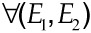: universal quantification. An instance ofoccurs whenever instances of both E1 and E2 have taken place, regardless of their order. That is, the hypomenonoccurs at the occurrence of the last of the hypomena El and E2 .

Other operations can be defined based on these basic ones. Some examples are:

• The macro any (), defined as the disjunction of all atomic events (including the simple events detected outside the system).

• The precedence operator before(E1, E2) (El, ( (; ))) E2 . An instance of this event occurs whenever an instance of E1 occurs prior to an instance of E2.

• The first occurrence first(E) E ,(E; ) . An instance of this event occurs whenever the fist instance of E occurs.

• The limited universal quantifier m(E1, E2,...En) with mn is true whenever m of the events E1,E2,...En are verified. The limited universal quantifier can be defined as a disjunction of all the universal quantifiers that give rise to the desired combination, for instance:

2(E1,E2, E3) = (E1,E2)⋁∀(E2,E3)⋁∀(E1, E3)⋁∀(E1, E2, E3)

It is easy to show that all binary operators defined above are associative so that, in most cases, I will use the abbreviated form (E1 * E2 * * En) where * is any of the binary operators above in lieu of the more cumbersome (H1*(H2*( *Hn))).

##### Parameters

Event expressions have parameter expressions associated with them, and event instances have associated the corresponding parameter values. Let P = (α1: D1,...αn: Dn) be a space of named parameters, and let D = D1 ... Dn be the corresponding data type. Given an event expression E, the expression E.αi is the expression of the ith parameter of E, and given an instance e, the expression e.αi is the value of the parameter. I will assume that for each occurrence of an event, the values of its parameters will not change.

##### Denotational Semantics

This section considers the semantics of events in terms, essentially, of denotational semantics . That is, in this section I am not yet concerned with the event semantics that derives from the context model, but only with the clarification of the formal semantics of the event definition language discussed so far.

In order to define the denotational semantics of events, it is first necessary to define the notions of system state and that of valid event occurrences. Informally, each event type (either a primitive event or an event expression) has associated two sets: a set of all event occurrences since the beginning of time, and the set of valid event occurrences, that is, of those occurrences that can be used to compute an expression. The need to specify a set of valid occurrences should be clear from the following example. Assume that in the system there are two primitive events, a and b, as well as the composite event c = a;b . The events a and b are instantiated as in Figure 22.3.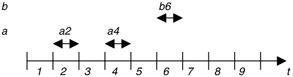Figure 22.3: The system with two primitive events.

When the event b6 is detected, the conditions are satisfied for the instantiation of the expression a; b, but how many such expression will be instantiated? There are two distinct sequences of events that could instantiate c, namely the sequence a2; b6 and the sequence a4; b6. Whether both these two sequences should be instantiated when b6 appears, only a2; b6 should be instantiated, or only a4; b6 rests on the definition of the semantics of the event expressions.

I will consider the semantics in terms of event histories. Each expression, once instantiated in a particular system, determines a function from the set of time instances to a co-domain in which it is possible to see whether at a specific time an event of that instance is occurring and what are the values of the parameters for that occurrence. The occurrence of an event is simply marked by a Boolean value, and the parameters are values of type D, as defined in the previous subsection. The whole status of an event at a given time is a value in 2 D1 Dn =2 D, where 2 is the data type of Boolean values. The fictitious parameter ω will be assumed to correspond to this component of the data type, so that the function e.ω is true when the event takes place.

Each primitive event and each event expression in the system determines an event type and, for each event type, a function [[E]]: T 2 D is defined. This function is the semantics of that event type. Primitive event types come with their semantics, given by the valid occurrences of the events and by the values of the parameters of these occurrences. Note that only the valid occurrences of events contribute to their semantics at a given time.

The set of semantics of all the events and event expressions in the system is the state of the event detection system. At any time t at which a new primitive event is instantiated, the new state of the system is determined in the following manner:

1. Determine the semantics of all the expressions in the system that may be affected by the new instantiation of the primitive event, computing a new function [[E]] for each of them.

2. Apply a state transition function, which depends on the specific semantics that is being used (see below) to the state thus obtained.

In order to define these state transition functions, it is necessary first to define another concept: that of minimally viable number of instance of an event a θ(a).This can be defined as the maximum number of consecutive instances of the event that needs to be preserved in order to be able to compute all the expressions in the system. Consider again the system above, with two sources of primitive events, a and b, and with a single expression: c = a;b . In this system, obviously, maintaining a single occurrence of a and a single occurrence of b will, in principle, allow one to compute the expression c. Let us, however, add a third expression d = (a b);a . One of the possible ways to instantiate this expression is by the sequence a2; a4. If we maintain a single occurrence of the event a in the state, we lose the possibility of detecting this sequence. So, in this case, it is θ(a) = 2.

With this concept in mind, I will define the following three state transition frameworks (these frameworks correspond, mutatis mutandis, to the Parameter contexts defined in ).

Recent: In this framework, only the most recent instances of the events necessary for the computation of an expression are used. Whenever an event of type E is instantiated, the state computation function will erase all the occurrences of the event except for the last θ(E). In the case of Figure 22.2, when the event a2 occurs, it will become part of the state. When a4 arrives, the instance a2 will be eliminated, and the event history of a will consist of the only instance a4. When b6 occurs, the event expression c will be evaluated on the current history consisting, at this time, of the instances a4 and b6, so that the instances that generates c will be a4; b6.

Chronological: In this framework, the oldest occurrence of an event that hasn't yet been used contributes to the computation of the expression that need it. The state transition function is composed of two parts: first, when a new primitive event is instantiated, the instance is added to the history of that event. Then, expressions are computed, and each one will use the oldest instances of the events that it needs, and mark them as used. Finally, after all the expressions have been computed, the state computation function will remove all marked instances, unless such removal would leave less than θ(E) instances.

In the example of Figure 22.2, the events a2 and a4 will be received and added to the history of the event type a, and similarly the event b6 will be added to the history of the event type b. At this point, the expression c will be evaluated, and it will make use of the instances a2 and b6, marking them as used. Then the state computation function will remove the instance a2 and b6, since they have already been used.

Continuous: In this framework, all the possible combinations that satisfy an expression are evaluated, and primitive events are discarded only after they have been used at least once. In the previous example, the construction of the state until the arrival of b6 proceeds as in the chronological framework but, when b6 arrives, both sequences a2; b6 and a4; b6 are used to instantiate two instances of the composite event c. After the instantiation, all the used instances that can be removed will be removed. In this case, all the events will be removed.

Absolute events derive their semantics from the interval in which they are defined, so, the semantics of the event [t1;t2] is the function

[[[t1; t2]]] = λt. (t tl t t2 )

Note that <.ω is true at any time the event is evaluated.

Operators, on the other hand, are maps from semantics to semantics. For instance, the conjunction operator A takes the semantics of two events (i.e., the functions determining their occurrences and the values of their parameters) and maps those into a function that is the semantics of the conjunction of the two events. In other words, the semantics of the conjunction operator is a map:

[[Λ]]: (T ) 2 Da) (T 2 Db) (T ) 2 D)

The semantics of the events used in the evaluation of a given operator will include, of course, only the instances that are valid at that particular time, the validity being determined by the framework that is being used.

I am making the usual compositionality assumption: the semantics of an expression involving an operator depends only on the semantics of the operator and on those of the events to which the operator is applied; in particular, the semantics of an expression is obtained by applying the operator semantics to the semantics of the events to which it is applied:

[[E1 E2]] = F([[]], [[ El ]], [[E2]]) = [[]]([[E1]], [[E2]])

Because of the definition of the parameter space each event has, roughly speaking, n+1 semantics, corresponding to the n+1 components of the data type 2 D: there is a function [[E]].ω: T 2 that determines the occurrence semantics of a given event expression (that is, it determines, given a certain event history, when the events specified by the event expression are taking place), and there are functions [[E]].αi : T Di that determine the parameter semantics of the event (that is, that compute, at any instant in which the event occurs, the pertinent value of the parameters). These functions are undefined when the event does not take place.

I will begin first by considering the occurrence semantics of the different expression, leaving the parameter semantics for the next section. In order to simplify the notation, I will omit the indication of the component from the semantic functions, writing [[E]] in lieu of [[E]].ω.

Finally, in order to facilitate the expression of the operator semantics, I will introduce two quantities: the last false time, and the last true time. The first quantity is defined for a time t only if, at t, the event expression that one is considering is true. It is, basically, the last time at which the event expression was false, and is defined as:

E(t) = t': [[E]](t') t",t' < t" t [[E]](t")

The last true time is the dual quantity: it is defined for a time t only if the expression under consideration is false, and is defined as the last time at which the expression was true:

E(t) = t': [[E]](t') t",t' < t" t [[E]](t")

As usual, only valid instances of the events according to the current state transition framework should be considered.

The semantics of the operators defined above is the following.

Sequence:The interpretation of this expression is the following: the sequence is true at the current time if the event E2 is true, the event E1 is false, and E2 became true immediately after E1 became false.

Relaxed sequence: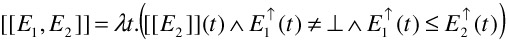In this case, the sequence is true at the current time if the event E2 is true and the event E2 became true after the event E1 became true. Note that the relaxed sequence event is true regardless of whether the event El is still happening when the event E2 happens or not. The diagram of Figure 22.4 shows some examples of the verification of a relaxed sequence (the dashed arrow points from an instance of E1 to the instance of the relaxed sequence that it enables).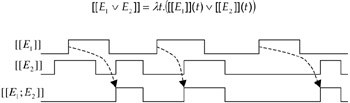Figure 22.4: Examples of the verification of a relaxed sequence.

Disjunction:

[[E1 E2]] = λt.([[E1]](t) [[E2]](t))

Conjunction:

[[E1 E2]] = λt. ([[E1]](t) [[E2]](t)

Negation:

[[ E]](t) = λt.( [[E]](t)

Universal Quantification:

[[(E1,E2)]] = λt.(t' t :[[E1]](t') [[E2]](t")

The definition of a formal semantics allows one to avoid confusion in the determination of the characteristics of an event expression. Using denotational semantics it is possible, for instance, to verify that the expression before is similar to the relaxed sequence but, unlike the latter, it occurs only when the event El finishes before E2 begins. That is, the second occurrence of the event [[E1;E2]] in Figure 22.3 would not take place if [[E1;E2]] were replaced by [[before(El,E2)]].

##### Parameter Semantics

Parameter semantics is strictly connected to the framework in use, because the framework will determine what instances of the events that form a given expression will be used to compute the parameters of the expression. The rules for which instances of an event will be used to compute the parameters of an expression are the same illustrated in the introduction to denotational semantics.

Unlike the occurrence semantics, however, the event operators do not induce any parameter semantics: the function that specifies the parameter of a complex event will have to be specified when the complex event is declared. This computation, however, must satisfy certain conditions, because it must be meaningful regardless the specific chain of events that leads to the instantiation of a complex event. For instance, a parameter of the expression (E1 E2) can't depend on a parameter that appears only in E1, because the event (E1 E2) can be instantiated even if E1 doesn't occur.

For an event E, let [[E]].P be the set of all its parameters. Parameters of two events E1 and E2 are considered equal if they have the same name and the same type. Then, the parameters of the event (E1 E2) will be computable only if they depend on the parameters in [[E1]].P [[E2]].P. That is, every parameter a of the disjunction of the two events must be defined as a function

[[E1 E2]].a = fa ([[El]].P [[E2]].P)

The set of parameters [[E1]].P [[E2]].P is called the computability set for the composite event (E1 E2). The computability set depends on the characteristics of the operators applied as indicated in Table 22.1.

Table 22.1

Expression

Computability set

(E1;E2)

[[E1]].P [[E2]].P

(E1;E2)

[[E1]].P [[E2]].P

(E1E2)

[[E1]].P[[E2]].P

(ElE2)

[[E1]].P[[E2]].P

E

(E1;E2)

[[E1].P[[E2]].P

##### Event Detection

There are two ways to proceed to event detection: one can either start from the earliest event that can appear in the instantiation of an expression, or from the last. Consider, for example, the expression E1;E2, and assume that E1 and E2 are primitive events.

One way of proceeding is to wait for an event of type E1 to occur and, when this event occurs, to put the system in a state that waits for E2 and whenever E2 arrives, detects the event E1; E2. This is tantamount to the definition of the state machine of Figure 22.5.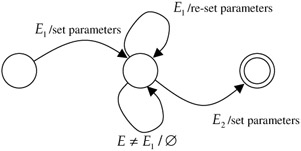Figure 22.5: State machine.

The complete state machine is more complicated, since it must take into account the different frameworks in which the computation is done. The state machine of Figure 22.5, since every new arrival of El causes the re-instantiation of the parameters, works in the recent framework.

A state machine like this can be easily defined for each operation, and state machines devoted to the detection of different complex events can be put together in a single push/pop machine to detect events of arbitrary complexity.

A second way of detecting complex events is to wait for the events that can conclude an expression. In the case of the expression E1; E2 this means waiting for the event E2. After this event has arrived, the system will look in the past history of events for a combination that will satisfy the whole expression. This way reduces event detection, essentially, to a regular expression search, but has the additional complication that a possibly unbounded history of past events must be kept.

I am well aware, of course, of the fact that there is no observing "reality as it is" through a camera. The very fact of the presence of a camera or, in the case in which the camera is concealed, its framing and de-contextualizing function, are sufficient to alter reality in a significant way. The "real reality" of which I am talking here should therefore be taken with a grain of salt, remembering always that what one observes through a camera is never the same as what one would have observed by being there.

The impossibility of formally defining context comes, in ultimate analysis, from the consideration that any formalism is, by itself, a meaningless play of symbols, and needs a context to be analyzed and to signify. The formalism in which a context would be defined, therefore, would require an additional context in order to be characterized, this context would require another one, and so on ad infinitum. There are, as far as I can see, only two ways of coming out of this impasse. The first is the aprioristic renounce to formally characterize a context. It is quite evident that this position, while philosophically the most satisfactory, is not sustainable in an engineering enterprise, in which the goal is to derive working systems, and an imperfect contextualization of the data is (with some caveats) better than no characterization at all. The second way, coherent with the engineering stance, is the acceptance of the limitations of any formalization of context. We know that our formalization will not be complete, nor do we expect it to be, and we know that this incompleteness will generate ambiguities and misinterpretations. The essential thing, in this case, is not to design systems as if the characterization of the context were complete, but to introduce safeguards, in terms of user interaction and interface devices, so that the ambiguities of the context will cause a graceful degradation of the usefulness of the system and not a dramatic breakdown.

The word hypomenon is, admittedly, a neologism. Its origin is in the Greek words hypo (under) and eim (I am).

The term simple is used here in reference to the event composition system presented here, of course, and it simply means that, from the point of view of the framework presented here, such events are to be regarded as indivisible and devoid of internal structure. Inside the system that detects them, on the other hand, the same event might very well have a structure and further components from which they are derived. These components are, however, assumed to be invisible in the current framework.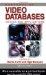Handbook of Video Databases: Design and Applications (Internet and Communications)
ISBN: 084937006X
EAN: 2147483647
Year: 2003
Pages: 393